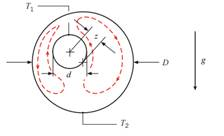### Create an Account

Already have account?

### Forgot Your Password ?

Home / Questions / Free convection heat transfer is sometimes quantified by writing Equation 420 as

# Free convection heat transfer is sometimes quantified by writing Equation 420 as

Free convection heat transfer is sometimes quantified by writing Equation 4.20 as qconv = Skeff  ∆T1-2, where keff is an effective thermal conductivity. The ratio keff/k is greater than unity because of fluid motion driven by buoyancy forces, as represented by the dashed streamlines.An experiment for the configuration shown yields a heat transfer rate per unit length of q’conv = 110 W/m for surface temperatures of T1 = 53°C and T2 = 15°C, respectively. For inner and outer cylinders of diameters d = 20 mm and D = 60 mm, and an eccentricity factor of z =10 mm, determine the value of keff. The actual thermal conductivity of the fluid is k = 0.255 W/m∙ K.

Jun 28 2020 View more View Less

#### Answer (Solved)Subscribe To Get Solution